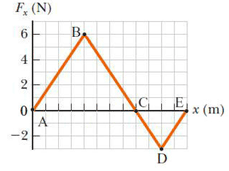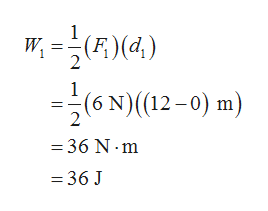Question
2 views

The force acting on a particle varies as in the figure below. (The x axis is marked in increments of 1.50 m.)

Find the work done by the force as the particle moves across the following distances.

(a) from
x = 0 m to x = 12.0 m

(b) from
x = 12.0 m to x=18.0m

(c) from
x = 0 m to x=18.0mhelp_outlineImage TranscriptioncloseF, (N) B. G E, x (m) -2 D fullscreen
check_circle

Step 1

(a)

The work done by the force as the particles moves from x=0 to x=12.0 m, that equals the area under graph, can be calculated as,help_outlineImage TranscriptioncloseW, =R)(4 =(6 N)(12-0) m) (F)(d.) = 36 N · m = 36 J fullscreen
Step 2

(b)

The work done by the force as the particles moves from x=12.0 m to x=18.0 m, tha...

### Want to see the full answer?

See Solution

#### Want to see this answer and more?

Solutions are written by subject experts who are available 24/7. Questions are typically answered within 1 hour.*

See Solution
*Response times may vary by subject and question.
Tagged in

### Kinematics